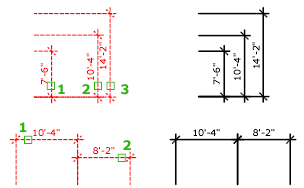# DIMSPACE (Command)

Adjusts the spacing between linear dimensions or angular dimensions.

Find

The spacing between parallel dimension lines is made equal. You can also make the dimension lines of a series of linear or angular dimensions line up by using a spacing value of 0.Spacing is applied only to parallel linear dimensions or to angular dimensions that share a common vertex.

The following prompts are displayed.

Select Base Dimension
Specifies a parallel linear or angular dimension from which the others will be spaced.
Select Dimensions to Space
Specifies parallel linear or angular dimensions to space equally from the base dimension. Press Enter after selecting all dimensions to be adjusted.
Enter Value
Applies a spacing distance between the selected dimensions.
Auto
Calculates the spacing distance automatically based on the text height specified in the dimension style of the selected base dimension. The resulting spacing distance is twice the height of the dimension text.